# Representing Entities as Sets Provides a Classical Explanation of Quantum Entanglement

Entanglement is usually described in terms of a physical theory, namely quantum theory (QT), wherein the entities formally represented in the theory are physical entities, e.g., electrons, photons, composites (ensembles) of any size. In contrast, I will describe entanglement in the context of an information processing model in which the entities formally represented in the theory are items of information. Of course, any item of information, even a single bit, is ultimately physically reified somewhere, even if only in the physical brain of a theoretician imagining the item. So, we might expect the two descriptions of entanglement, one in terms of a physical theory and the other in terms of an information processing theory, to coalesce, to become equivalent, cf. Wheeler’s “It from Bit”. This essay works towards that goal.

The information processing model used here is based on representing items of information as sets, specifically, (relatively) small subsets, of binary representational units chosen from a much larger universal set of these units. This type of representation has been referred to as a sparse distributed representation (SDR) or sparse distributed code (SDC). We’ll use the term, SDC. Fig. 1 shows a small toy example of an SDC model. The universal set, or coding field (or memory), is organized as a set of Q=6 groups of K=7 binary units. These groups function in winner-take-all (WTA) fashion and we refer to them as competitive modules (CMs). The coding field is completely connected to an input field of binary units, organized as a 2D array (e.g., a primitive retina of binary pixels), in both directions, a forward matrix of binary connections (weights) and a reverse matrix of binary weights. All weights are initially zero. We impose the constraint that all inputs have the same number of active pixels, S=7.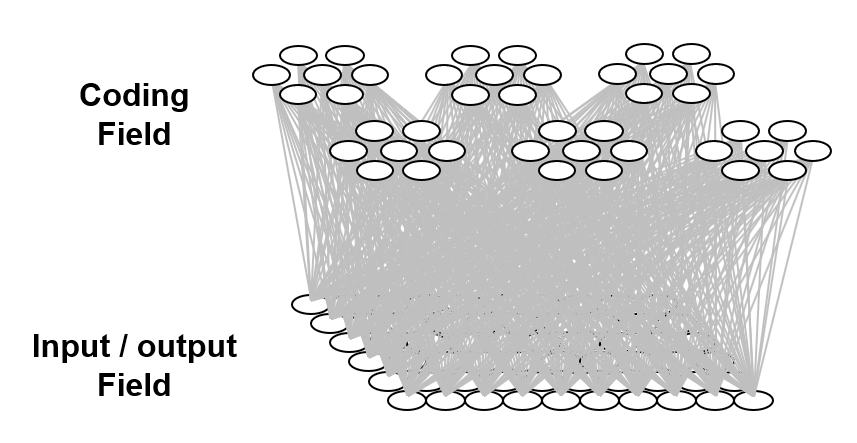Fig. 1: Small example of an SDC-based model. The coding field consists of Q=6 competitive modules (CMs), each consisting of K=7 binary units. The input field is a 2D array of binary units (pixels) and is completely connected in both directions, i.e., a forward and a reverse matrix of binary weights (grey lines, each on represents both the forward and the reverse weight).

The storage (learning) operation is as follows. When an input item, Ii, is presented (activated in the input field), a code, φ(Ii) consisting of Q units, one in each of the Q CMs, is chosen and activated and all forward and reverse weights between active input and active coding field units are set to one. In general, some of those weights may already be one due to prior learning. We will discuss the algorithm for choosing the codes during learning in a later section. For now, we can assume that codes are chosen at random. In general, any given coding, α, unit will be included in the codes of multiple inputs. For each new input in whose code α is included, if that input includes units (pixels) that were not active in any of the prior inputs in whose codes α was included, the weights from those pixels to α will be increased. Thus, the set of input units having a connection with weight one onto α can only increase with time (with further inputs). We call that set of input units with w=1 onto α, α’s tuning function (TF),

The retrieval operation is as follows. An input, i.e., a retrieval cue, is presented, which causes signals to travel to the coding field via the forward matrix, whereupon the coding field units compute their input summations and the unit with the max sum in each CM is chosen winner, ties broken at random. Once this “retrieved” code is activated, it sends signals via the reverse matrix, whereupon, the input units compute their input summations and are activated (or possibly deactivated) depending on whether a threshold is exceeded. In this way, partial input cues, i.e., with less than S active units, can be “filled in” and novel cues that are similar enough to previously (learned) inputs can cause the codes of such, closest-matching learned inputs to activate, which can then cause (via the reverse signals) activation of that closest-matching learned input.

With the above description of the model’s storage and retrieval operations in mind, I will now describe the analog of quantum entanglement in this classical model. Fig. 2 shows the event of storing the first item of information, I1, into this model. I1 consists of the S=7 light green input units. The Q=6 green coding field units are chosen randomly to be I1‘s code, φ(I1), and the black lines show some of the weights that would be increased, from 0 to 1, in this learning event. Each line represents both the forward and reverse weight connecting the two units. More specifically, the black lines show all the forward/reverse weights that would be increased for two of φ(I1)’s units, denoted α and β. Note that immediately following this learning event (the storage of I1), α and β have exactly the same TF. In fact, all six units comprising φ(I1) have exactly the same TF. In the information processing realm, and more pointedly in neural models, we would describe these six units as being completely correlated. That is, all possible next inputs to the model, a repeat presentation of I1 or any other possible input, would cause exactly the same input summation to occur in all six units, and thus, exactly the same response by all six units. In other words, all six units carry exactly the same information, i.e., are completely redundant.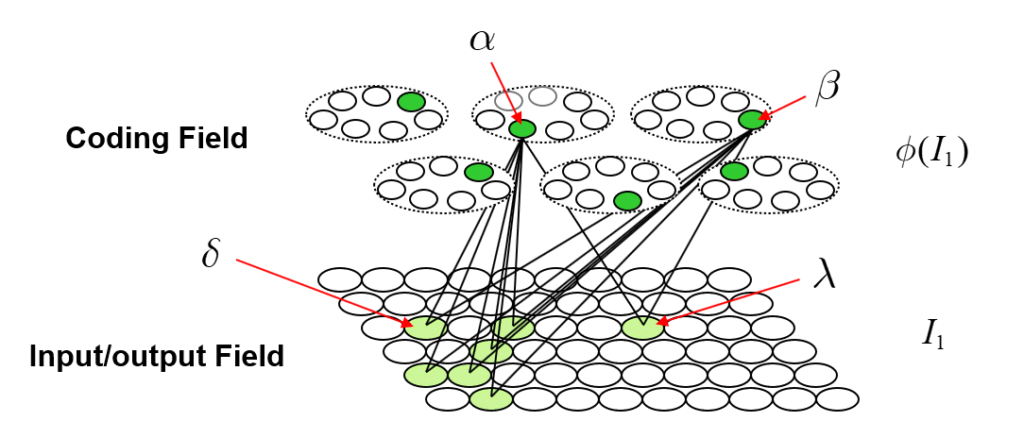Fig. 2: The first input to the model, I1, consisting of the S=7 light green units, has been presented and a code, φ(I1), consisting of Q=6 units (green), has been chosen at random. All forward and reverse weights between active input and coding fields units are set to one. The black lines show all such weights involving coding units α and β.

As the reader may already have anticipated, I will claim that these six units are completely entangled. We can consider the act of assigning the six units as the code, φ(I1), as analogous to an event in which a particle decays, giving rise, for example, to two completely entangled photons. In the moment when the two particles are born out of the vacuum, they are completely entangled and likewise, in the moment when the code, φ(I1), is formed, the six units comprising it are completely entangled. However, in the information processing model described here, the mechanism that explains the entanglement, i.e., the correlation, is perfectly clear: it’s the correlated increases to the forward/reverse weights to/from the six units that entangles those units. Note in particular, that there are no direct physical connections between coding field units in different CMs. This is crucial: it highlights the fact that in this explanation of entanglement, the hidden variables are external, i.e., non-local, to the units that are entangled. The hidden variables ARE the connections (weights). This hints at the possibility that the explanation of entanglement herein comports with Bell’s theorem. The 2015 results proving Bell’s theorem, explicitly rule out only local hidden variables explanations of entanglement. Fig. 3 shows the broad correspondence between the information theory and the physical theory proposed here.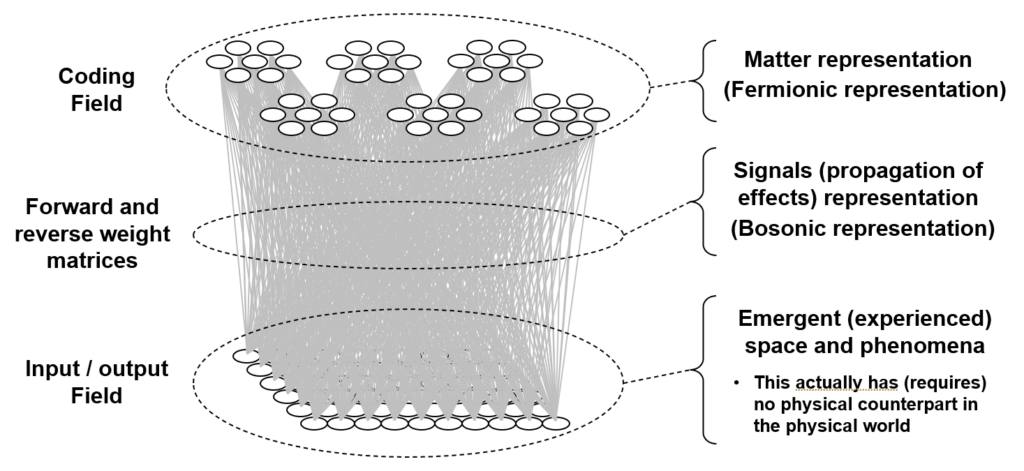Fig. 3: Broad correspondence between the information processing theory described here (and previously throughout my work) and the proposed physical theory. This figure will generalized in a later section to include the recurrent matrix that connects the coding field to itself (and is also part of the bosonic representation), which is needed to explain how the physical state represented by the coding field is updated through time, i.e. the dynamics.

Suppose we now activate just one of the seven input units comprising I1, say δ. Then φ(I1) will activate in its entirety (i.e., all Q=6 of its units) due to the “max sum” rule stated above, and then the remaining six input units comprising I1 will be activated based on the inputs via the reverse matrix. Suppose we consider the activation of δ to be analogous to making a measurement or observation. In this interpretation, the act of measuring at one locale of the input field, at δ, immediately determines the state of the entire coding field and subsequently (via the reverse projection) the entire state of the input field [or more properly, input/output (“I/O”) field], in particular, the state of the spatially distant I/O unit, λ. Note that there is no direct connection between δ and λ. The causal and deterministic signaling went, in one hop, via the connections of the forward and reverse matrices. Thus, while our hidden variables, the connections, are strictly external (non-local) to the units being entangled (i.e., the connections are not inside any of the units, either coding units or I/O units), they themselves are local in the sense of being immediately adjacent to the entangled units. Thus, this explanation meets the criteria for local realism. This highlights an essential assumption of my overall explanation of quantum entanglement. It requires that physical space, or rather the underlying physical substrate from which our apparent 3D space and all the things in it emerge, be composed of smallest functional units that are completely connected. In another essay, I call these smallest functional, or atomic functional, units, “corpuscles”. Specifically, a corpuscle is comprised of a coding field similar to, but vastly larger than (e.g., Q=106, K=1015), those depicted here and: a) a recurrent matrix of weights that completely connects the coding field to itself; and b) matrices that completely connect the coding field to the coding fields in all immediately neighboring corpuscles. In particular, the recurrent matrix allows the state of the coding field at T to influence, in one signal-propagation step, the state of the coding field at T+1. Thus, to be clear, the explanation of entanglement given here applies within any given corpuscle. It allows instantaneous, i.e., faster than light, transmission of effects throughout the region of (emergent) space corresponding to the corpuscle, which I’ve proposed elsewhere to be perhaps 1015 Planck lengths in diameter. If we define the time, in Planck times, that it takes for the corpuscle to update its state to equal the diameter of corpuscle, in Planck lengths, then signals (effects) are limited to propagating across larger expanses, i.e., from one corpuscle to the next, no faster than light.

Here I must pause to clarify the analogy. In the typical description of entanglement within the context of the standard model (SM), it is (typically) the fundamental particles of the SM, e.g., electrons, photons, which become entangled. In the explanation described here, it is the individual coding units that become entangled. But I do not propose that that these coding field units are analogous to individual fundamental particles of the standard model (SM). Rather, I propose that the fundamental particles of the SM correspond to sets of coding field units, in particular, to sets of size smaller than a full code, i.e., smaller than Q. That is, the entire state of region of space emerging from a single corpuscle is a set of Q active coding units. But a subset of those Q units might be associated with any particular entity (particle) present in that region at any given time. For example, a particular set of coding units, say of size Q/10, might always be active whenever an electron is present in the region, i.e., that “sub-code” appears as a subset in all full codes (of size, Q) in which an electron is present. Thus, rather than saying that, in the theory described here, individual coding units become entangled, we can slightly modify it to say that subsets of coding units become entangled.

Returning to the main thread, Fig. 4 now describes how patterns of entanglement generally change over time. Fig. 4a is a repeat of Fig. 2, showing the model after the first item, I1, is stored. Fig. 4b shows a possible next input, I2, also consisting of S=7 active I/O units, one of which, δ is common to I1. Black lines depict the connections whose weights are increased in this event. The dotted line is to indicate that the weights between α and δ will have been increased when I1 was presented. Fig. 4c shows α’s TF after I2 was presented. It now includes the 13 I/O units in the union of the two input patterns, {I1 ∪ I2}. In particular, note that coding units, α and β are no longer completely correlated, i.e., their TFs are no longer identical, which means that we could find new inputs that elicit different responses in α and β. In general, the different responses, can lead, via reverse signals, to different patterns of activation in the I/O field, i.e., different values of observables. In other words, they are no longer completely entangled. This is analogous to two particles which arise completely entangled from a single event, going off and having different histories of interactions with the world. With each new interaction that either one has, they become less entangled. However, note that even though α and β are no longer 100% correlated, we can still predict either’s state of activation based on the other’s at better-than-chance accuracy, due to the common units in their TFs and to the corresponding correlated changes to their weights.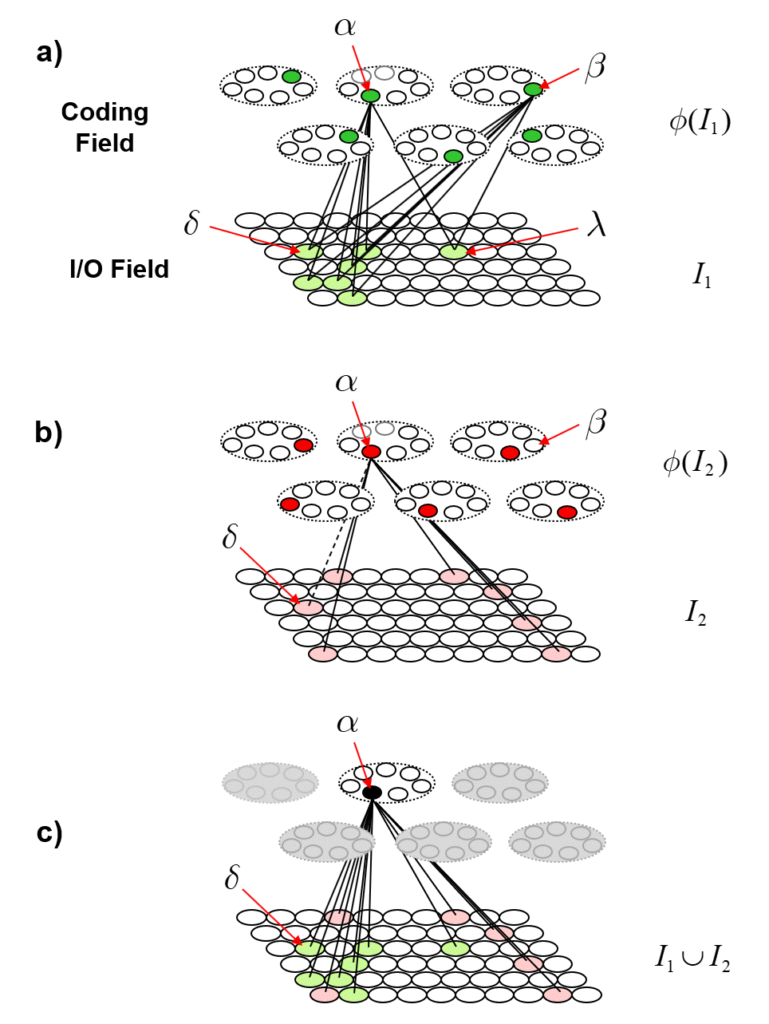Fig. 4: (a) Repeat of Fig. 2 for reference. (b) Presentation of the second input, I2, its randomly selected code, φ(I2), and the new learning involving coding unit α. The weights to/from I/O unit δ are dashed since they were already increased to one when I1 was presented, i.e., δ was active in both inputs. (c) α’s TF is the union of all I/O units that are active in any input in whose code α was included. In particular, α and β are no longer completely entangled (correlated), due to this additional interaction α has had with the world.

Fig. 5 now shows a more extensive example illustrating the further evolution of the same process shown in Fig. 4. In Fig. 5, we show coding unit α participating in a further code, φ(I3), for input I3. And we show coding unit β participating in the codes of two other inputs, I4 and I5, after participating, along with α, in the code of the first input, I1. Panel d shows the final TF for α after being used in codes of three inputs, I1, I2 and I3. Panel g shows the final TF for β after being used in the codes for I1, I4 and I5. One can clearly see how the TFs gradually diverge due to their diverging histories of usage (i.e., interaction with the world). This corresponds to α and β becoming progressively less entangled with successive usages (interactions).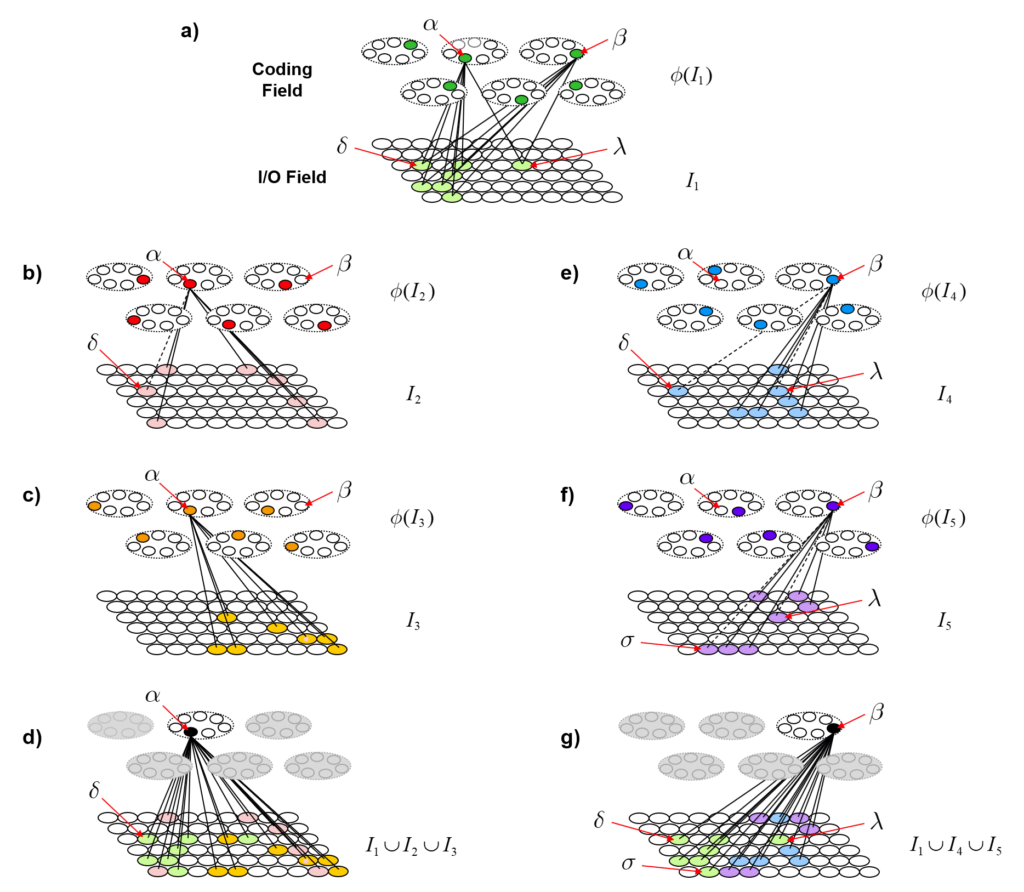Fig. 5: Depiction of the continued diminution of the entanglement of α and β as they participate in different histories of inputs. Panel d shows the final TF for α after being used in codes of three inputs, I1, I2 and I3. Panel g shows the final TF for β after being used in the codes for I1, I4 and I5.

To quantify the decrease in entanglement precisely, we need to do a lot of counting. Fig. 6 shows the idea. Figs. 6a and 6b repeat Figs. 5d and 5g, showing the TFs of α and β. Figs. 6c and 6d show the intersection of those two TFs. The colored dots indicate the input from which the particular I/O unit (pixel) was accrued into the TF. In order for any future input [including any repeat of any of the learned (stored) ones] to affect α and β differently (i.e., to cause α and β to have different input sums, and thus, to have different chances of winning in their respective CMs, and thus, to ultimately cause activation of different full codes), that code must include at least one I/O unit that is in either TF but not in the intersection of the TFs. Again, all inputs are constrained to be of size S=7. Thus, after presenting any input, we can count the number of such codes that meet that criterion. Over long histories, i.e., for large sets of inputs, that number will grow with each successive input.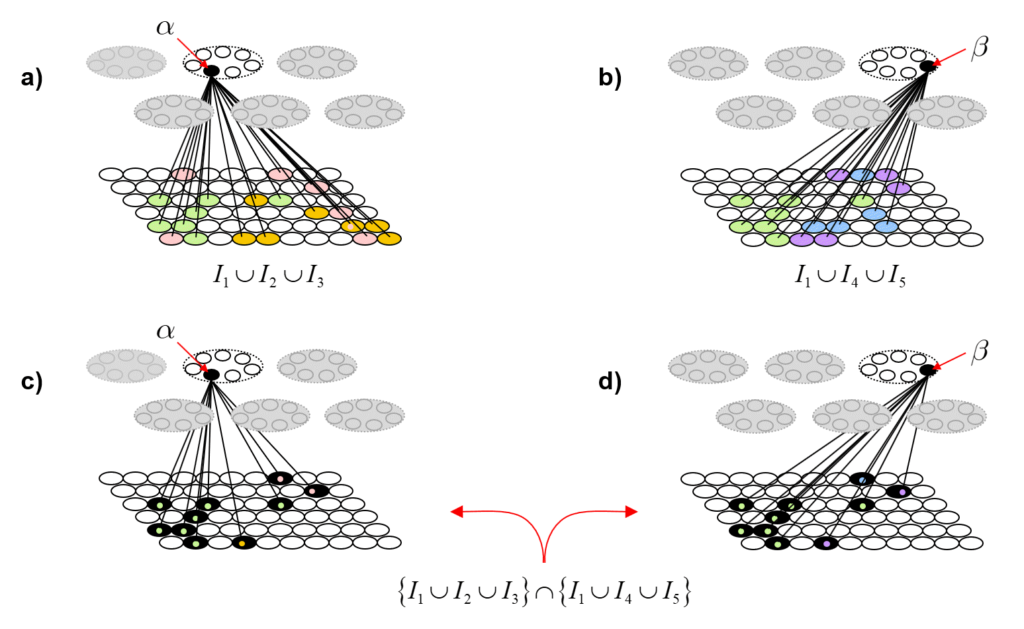Fig. 6: (a,b) Repeats of Figs. 5d and 5g showing the TFs of α and β. (c,d) The union of the two TFs (black cells). The colored dots tell from which input the I/O unit (pixel) was accrued into the relevant coding unit’s TF.

## A storage (learning) algorithm that preserves similarity

In the examples above, the codes were chosen randomly. While we can and did provide an explanation of entanglement under that assumption, a much more powerful and sweeping physical theory emerges if we can provide a tractable mechanism (algorithm) for choosing codes in a way that statistically preserves similarity, i.e., maps similar inputs to similar codes. In this section, I will describe that algorithm. The final section will then revisit entanglement, providing further elaboration.

# A Simple explanation of the Holographic Principle

The holographic principle says that the maximum amount of information that can be contained in a volume (bulk) equals the amount of information that can be contained on the surface of that bulk. On first thought, this seems absurd: Common sense tells us that there is so much more matter comprising a bulk than comprising its surface. Yet, this is what the work of ‘t Hooft and others tells us. Fig. 1 (from ‘t Hooft’s 2000 paper, “The Holographic Principle”) shows a tiling in which each patch, of area equal to 4 x Planck area (~4 x 10-70 sq. m.), can hold one bit of information. Since black holes are the most dense objects, we can take this number, the area of the surface of a spherical volume in units of Planck areas divided by 4 to be an upper bound on the number of bits that can be stored in that volume. In fact, the explanation given in this essay suggests the upper bound is just slightly less than the area in Planck units.Fig. 1: Linked from Gerard ‘t Hooft’s “The Holographic Principle” (2000). Depiction of the result that the number of bits of information contained in a black hole equals the area of the black hole’s surface in Planck areas divided by 4.

If the Planck length is the smallest unit of spatial size, then 2D surfaces in space have areas that are discretely valued and 3D regions have volumes that are discretely valued. Since the cube is the the only regular and space-filling polyhedron for 3D space, we therefore assume that space is a cubic tiling of Planck volumes as in Fig. 2. If the Planck volume is the smallest possible volume then it can have no internal structure. Thus, the simplest possible assumption is that it is binary-valued; either something exists in that volume (“1”) or not (“0”). I call these Planck-size volumes, “Planckons”. However, note that despite the ending “ons”, these are not particles like those of the Standard Model (SM). In particular, they do not move: they are the units of space itself.

I contend that that the this assumption, that space is a 3D tiling of binary-valued Planck cubes already explains the why the amount of information that can be contained in a volume is upper-bounded by the amount of information that can be contained on its surface, i.e., the holographic principle. For example, consider a cube-shaped volume of space, i.e., a bulk, that is 8 Planck lengths on each side, as shown in Fig. 3 (rose colored Planckons). This bulk, consists of 83 = 512 Planckons. Consider the layer of Planckons that forms the immediate boundary of this bulk. We can take the boundary to be just the union of the six 2D arrays of Planckons that are immediately adjacent to the six faces. Thus the boundary consists of 6 x 82 = 384 Planckons. In the case of a black hole, this boundary is the event horizon. The reason why we ignore the Planckons that run along just outside the edges of the bulk and those at the corners just outside the bulk is that we assume that physical effects can only transmit through faces of Planckons.. The boundary constitutes a channel through which all physical effects, i.e., communication (information), to and from this bulk must pass. The number of unique states that the bulk can be in is 2512. On first glance, this suggests, that the amount of information that can be stored in this bulk is log22512bits, i.e., 512 bits. The number of unique states of the channel is only 2384, suggesting it can hold only log22384 = 384, bits of information. In fact, the holographic principle says that the number of bits storable in the channel is only 384 / 4 = 96 bits. However, the explanation developed here suggests the limit is just less than 384. The reason it’s not exactly equal to to 384 is a slight diminution due to edge and corner effects. In any case, the overall argument explains why the information storage capacity is determined by the area of the surface bounding a volume and not by the volume.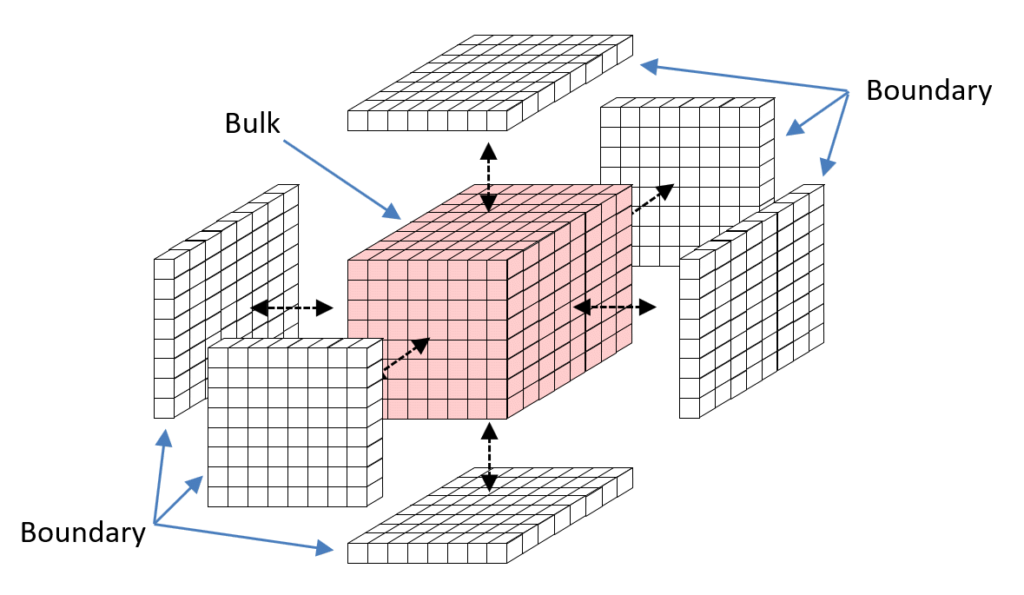Fig.3: Depiction of a 3D bulk consisting of 8x8x8=512 binary Planckons and its boundary that is the union of the six 8×8 2D arrays of Planckons, for a total of 384 Planckons, that wraps the bulk.

Try to imagine possible mechanisms by which the states of Planckons submerged in the bulk could be accessed, i.e., read or written. Any such infrastructure and mechanism for implementing such read or write operations would have to reside in the bulk. E.g., perhaps one might imagine some internal system of say, 1-Planckon thick, buses threading through the bulk, providing access to those submerged Planckons. Thus, some of the 512 bulk Planckons cannot be available for storing information. While this would reduce the information storage limit to something less than the full volume in Planck volumes, it does not explain why it should be reduced all the way down to being approximately equal the number of Planckons comprising the 2D boundary.

Rather than address the 3D case directly, let’s reduce the dimension of the problem by one. Accordingly, Fig. 4 shows a 2D space, a 2D bulk, the 5×5 array of rose Planckons, wrapped by a 1D boundary, comprised of 20 white Planckons. The analogy of the 3D version of the holographic principle would predict that the amount of information that can be stored in the 25 Planckon (25 bit) bulk equals the amount of information that can be stored in the 20 Planckon boundary, again, disregarding the divisor of four.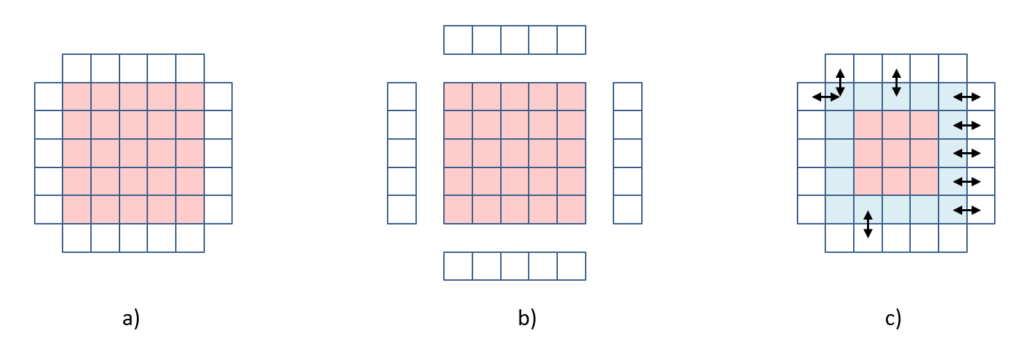Fig. 4: (a,b) a 2D bulk (red square) consisting of 5×5 Planckons, surrounded by a 1D boundary of Planckons. (c) The 16 light blue Planckons of the bulk are in direct contact with the boundary. Assuming that physical (causal) effects, i.e., signals, can only be transmitted through shared edges, not corners, then only these 16 bulk Planckons can be written or read from (through) the boundary.

Since we assume that physical effects can only be transmitted through edge connections (not corners), only the 16 bulk Planckons comprising the outermost, one-Planckon-thick shell of the bulk (blue Planckons) are directly accessible from the boundary (arrows show examples of access paths). We can imagine those 16 bulk Planckons, as a memory of bits just as in a regular computer memory and the Planckons comprising the boundary (perhaps along with additional Planckons extending into the space beyond the boundary) to be a bus, or connection matrix, for accessing (writing or reading) these 16 bits. In any case, the innermost nine Planckons of the bulk (rose Planckons in Fig. 4c) are not directly accessible from the boundary. We then have the question as to how the states of those innermost nine bulk Planckons could help to increase the storage capacity of the bulk.

Rather than attempt to construct such an infrastructure and (read and write) operations, let’s instead imagine how submerged Planckons might be able to be seen through other Planckons, and thus possibly affect the state of the (one or more) boundary Planckons. We can actually make the problem easier, and without loss of generality, by reducing the problem by one dimension.

The answer is that they cannot increase the storage capacity. Why?

It seems there are two approaches

• We can treat the bulk as a cellular automata in which case we define a rule by which a Planckon updates its states as a function of its own state and those of its four edge-adjacent neighbors. In this case, there is only one rule, which operates in every Planckon. In particular, this means all Planckons are of the same type.
• We can treat consider the bulk’s Planckons as being of two types: a) ones that hold a binary state, i.e., bits of memory, as we have already postulated for the outermost 16 Planckons of the bulk; and b) ones that implement communication, i.e., transmit signals, in which case, we’re allowing that the communication infrastructure (bus, matrix) now extends into the bulk itself.

In the first approach, for there to be a positive answer to the challenge question, there must be an update rule (i.e. dynamics) which allows 25 bits to be stored and retrieved. In the second approach, where we partition the bulk’s Planckons into two disjoint subsets, those that hold state, which we will call a memory, or a coding field, and those that transmit signals, we need to define the structure (topology) of both parts, the coding field and the signal transmission partition.

…STILL BEING WRITTEN…

So it seems that we are led to the concept of a corpuscle of space. As defined elsewhere, the corpuscle is the smallest completely connected region of space, i.e., where the coding field Planckons are completely connected to themselves (a recurrent connection) and completely connected to the Planckons comprising the corpuscle’s boundary. So in this case, the model is explicitly not a cellular automata, where the physical units tiling a space are connected only to nearest neighbors according to the dimension of the space in which the physical units exist. Rather, by assigning some Planckons to be for communication and some for states, we make possible connectivity schemes that are not limited to the underlying space’s dimension, but rather can allow arbitrarily higher dimensionality (topology) for subset of Planckons devoted to representing state.

So it’s just as simple as that. If space is discrete, then the amount of information that can be contained in a 3D volume equals the amount of information that can be contained in its 2D (though actually, one-Planckon thick) boundary. Also, note that the argument remains qualitatively the same if we consider the bulk and boundaries as spheres instead.

There are only 2384 possible messages (signals) we could receive from this bulk. So even though there are vastly more , i.e., 2512, unique states of the bulk, all of those states necessarily fall into only 2384 equivalence classes. And similarly, there are only 2384 messages we could send into the bulk, meaning that all possible states of the vastly larger world outside the bulk similarly fall into only 2384 equivalence classes. No matter what computational process we can imagine that operates inside the bulk, i.e., no matter which of it’s 2384 states is produced by such process, and furthermore, no matter how many steps the process producing that state takes, it can only produce 2384 output messages.

A well-known quantum computation theorist told me that the above is simply a re-statement of the holographic principle, not an explanation. In particular, he said that I need to explain why we could not send more than 384, or in fact, all 512 bits of information into the bulk by sending multiple messages. So here is the explanation of why multiple messages doesn’t help.

# Learned Multidimensional Indexes

At the ends of papers and talks on the “learned indexes” concept described by Kraska et al in 2017, an exciting future research direction referred to as “multidimensional indexes” is suggested.

I described a multidimensional index concept about 10 years ago (unpublished) but referred to it as learning representations that were “simultaneously physically ordered on multiple uncorrelated dimensions”. The crucial property that allows informational items, e.g., records of a database consisting of multiple fields (dimensions, features), to be simultaneously ordered on multiple dimensions is that they have the semantics of extended bodies, as opposed to point masses.  Formally, this means that items must be represented as sets, not vectors.

Why is this? Fig. 1 gives the immediate intuition. Let there be a coding field consisting of 12 binary units and let the representation, or code, which we will denote with Greek letter φ, of an item be a subset consisting of 5 of the 12 units.  First consider Fig. 1a.  It shows a case where three inputs, X, Y, and Z, have been stored.  To the right of the codes, we show the pairwise intersections of the three codes.  In this possible world, PW1, the code of X is more similar to the code of Y than to that of Z.  We have not shown you the specific inputs and we have not described the learning process that mapped these inputs to these codes.  But, we do assume that that learning process preserves similarity, i.e., it maps more similar input to more highly intersecting codes.  Given this assumption, we know that

sim(X,Y) > sim(X,Z) and also that sim(Y,Z) > sim(X,Z).

Fig. 1

That is, this particular pattern of code intersections imposes constraints on the statistical (and thus, physical) structure of PW1.  Thus, we have some partial ordering information over the items of PW1. We don’t know the nature of the physical dimensions that have led to this pattern of code intersections (since we haven’t shown you the inputs or the input space).  We only know that there are physical dimensions on which items in PW1 can vary and that that X, Y, and Z have the relative similarities, relative orders, given above.  But note that given only what has been said so far, we could attach names to these underlying physical dimensions (regardless of what they actually are).  That is, there is some dimension of the input space on which Y is more similar to X than is Z.  Thus, we could call this dimension, “X-ness”.  Y has more X-ness than Z does.  Similarly, there is another physical dimension present that we can call “Y-ness”, and Z has more Y-ness than X does.  Or, we could label that dimension “Z-ness”, in which case, we’d say that Y has more Z-ness than X does.

Now, consider Fig 1b.  It shows an alternative set of codes for X, Y and Z, that would result if the world had a slightly different physical structure.  Actually, the only change is that Y has a slightly different code.  Thus, the only difference between PW2 and PW1 is that in PW2, whatever physical dimension X-ness corresponds to, item Y has more of it than it does in PW1. That’s because |{φ(X) ∩ φ(Y)| = 3 in PW2, but equals 2 in PW1. ALL other pairwise relations are the same in PW2 as they are in PW1.  Thus, what this example shows, is that the representation has the degrees of freedom to allow ordering relations on one dimension to vary without impacting orderings on other dimensions. While it is true that this is a small example, I hope it is clear that this principle will scale to higher dimensions and much larger numbers of items.  Essentially, the principle elaborated here leverages the combinatorial space of set intersections (and intersections of intersections, etc.) to counteract the curse of dimensionality.

The example of Fig. 1 shows that when items are represented as sets, they have the internal degrees of freedom to allow their degrees of physical similarity on one dimension to be varied while maintaining their degrees of similarity on another dimension.  We actually made a somewhat stronger claim at the outset, i.e., that items represented as sets can simultaneously exist in physical order on multiple uncorrelated dimensions.  Fig. 2 shows this directly, for the case where the items are in fact simultaneously ordered on two completely anti-correlated dimensions.

In Fig. 2, the coding field consists of 32 binary units and the convention is that all codes stored in the field will consist of exactly 8 active units. We show the codes of four items (entities), A to D, which we have handpicked to have a particular intersection structure.  The dashed line shows that the units can be divided into two disjoint subsets, each representing a different “feature” (latent variable) of the input space, e.g., Height (H) and IQ (Q).    Thus, as the rest of the figure shows, the pattern of code intersections simultaneously represents both the order, A > B > C > D, for Height and the anti-correlated order, D > C > B > A, for IQ.

Fig. 2

The units comprising this coding field may generally be connected, via weight matrices, to any number of other, downstream coding fields, which could “read out” different functions of this source field, e.g., access the ordering information on either of the two sub-fields, H or Q.

The point of these examples is simply to show that a set of extended objects, i.e., sets, can simultaneously be ordered on multiple uncorrelated dimensions.  But there are other key points including the following.

1. Although we hand-picked the codes for these examples, the model, Sparsey, which is founded on using a particular format of fixed-size sparse distributed representation (SDR), and which gave rise to the realization described in this essay, is a single-trial, unsupervised learning model that allows the ordering (similarity) relations on multiple latent variables to emerge automatically.  Sparsey is described in detail in several publications: 1996 thesis, 2010, 2014, 2017 arxiv.
2. While conventional, localist DBs use external indexes (typically trees, e.g., B-trees, KD-trees) to realize log time best-match retrieval, the set-based representational framework described here actually allows fixed-time (no serial search) approximate best-match retrieval on the multiple, uncorrelated dimensions (as well as allowing fixed-time insertion).  And, crucially, there are no external indexes: all the “indexing” information is internal to the representations of the items themselves.  In other words, there is no need for these set objects to exist in an external coordinate system in order for the similarity/ordering relations to be represented and used.

Finally, I underscore two major corollary realizations that bear heavily on understanding the most expedient way forward in developing “learned indexes”.

1. A localist representation cannot be simultaneously ordered on more than one dimension.  That’s because localist representations have point mass semantics.  All commercial DBs are localist: the records of a DB are stored physically disjointly.  True, records may generally have fields pointing to other records, which can therefore be physically shared by multiple records.  But any record must have at least some portion that is physically disjoint from all other records.  The existence of that portion implies point mass semantics and (ignoring the trivial case where two or more fields of the records of a DB are completely correlated) a set of points can be simultaneously ordered (arranged) on at most one dimension at a time.  This is why a conventional DB generally needs a unique external index (typically some kind of tree structure) for each dimension or tuple on which the records need to be ordered so as to allow fast, i.e., log time, best-match retrieval.
2. In fact, dense distributed representations (DDR), e.g., vectors of reals, as for example present in the internal fields of most mainstream machine learning / deep learning models, also formally have point mass semantics.  Intersection is formally undefined for vectors over reals.  Thus, any similarity measure between vectors (points) must also formally have point mass semantics, e.g., Euclidean distance.  Consequently, DDR also  precludes simultaneous ordering on multiple uncorrelated dimensions.

Fig. 3 gives final example showing the relation of viewing items in terms of a point representation to set representation.  Here the three stored items are purple, blue, and green.  Fig. 3a begins showing the three items as points with no internal structure sitting in a vector space and having some particular similarity (distance) relationships, namely that purple and blue are close and they are both  far away from green.  In Fig. 3b, we now have set representations of the three items.  There is one coding field here, consisting of 6×7=42 binary units and red units show intersection with the purple item’s representation. Fig 3c shows that the external coordinate system is no longer needed to represent the similarity (distance) relationships, and Fig. 3d just reinforces the fact that there is really only one coding field here and that the three codes are just different activation patterns over that single field. The change from representing information formally as points in an external space to representing them as sets (extended bodies) that require no external space will revolutionize AI / ML.

Fig. 3

# Not Copenhagen, Not Everett, a New Interpretation of Quantum Reality

I’ve claimed for some time that quantum computing can be realized on a single processor Von Neumann machine and published a paper explaining the basic intuition. All that is necessary is to represent information using sparse distributed codes (SDC) rather than localist codes. With SDC, all informational states (hypotheses) represented in a system (e.g., computer) are represented in physical superposition and therefore all such states are partially active in proportion to their similarity with the current most likely (most active) state. My Sparsey® system operates directly on superpositions, transforming the superposition over hypotheses at T into the superposition at T+1 in time that does not depend on the number of hypotheses represented (stored) in the system. This update occurs via passage of signals via a recurrent channel, i.e., a recurrent weight matrix. In addition to a serial (in time) updating of the superposition, mediated by the recurrent matrix, the system may also have an output channel (a different, non-recurrent weight matrix) that “taps” off, i.e., observes, the superposition at each T. Thus the system can generate a sequence of collapsed outputs (observations) which can be thought of as a sequence of maximum likelihood estimates, as well as continuously update the superposition (without requiring collapse).

This has led me to the following interpretation of the quantum theory of the physical universe itself.  Projecting a universe of objects in a low dimensional space, e.g., 3 dimensions, up into higher dimensional spaces, causes the average distance between objects to increase exponentially with the number of dimensions. (The same is true of sparse distributed codes living in a sparse distributed code space.) But now imagine that the objects in the low dimensional space are not point masses, but rather have extension. Specifically, let’s imagine that these objects are something like ball-and-stick lattices, or 3D graphs consisting of edges and nodes. The graph has extension in 3 dimensions, but is mostly just space.  Further, imagine that the graph edges simply represent forces between the nodes (and not constrained to be pairwise forces), where the nodes are the actual material constituents of objects (similar to how an atom is mostly space…and perhaps even a proton is mostly empty space).

Now suppose that the actual universe is of huge dimension, e.g., a million dimensions, or an Avogadro’s number of dimensions, but let’s stick with one million for simplicity. Furthermore, imagine that these are all macroscopic dimensions (as opposed to the Planck-scale rolled up dimensions of string theory). Now imagine that this million-D universe is filled with macroscopic “graph” objects.  They would have macroscopic extent on perhaps a large fraction or even all of those 1 million dimensions, but they would be almost infinitely sparse or diffuse, i.e., ghost-like, so diffuse that numerous, perhaps exponentially numerous such objects, could exist in physical superposition with each other, i.e., physically intermingled.  They could easily pass through each other.  But, as they did so, they would physically interact.

Suppose that we can consider two graphs to be similar in proportion to how many nodes they share in common. Thus two graphs that had a high fraction of their nodes in common might represent two similar states of the same object.

But suppose that instead of thinking of a single graph as representing a single object, we think of it as representing a collection of objects.  In this case, two graphs having a certain set of nodes in common (intersection), could be considered to represent similar world states in which some of the same objects are present and perhaps where some of the those objects have similar internal states and some of the inter-object relations are similar.  Suppose that such a graph, S, consisted of a very large number (e.g., millions) of nodes and that a tiny subset, for concreteness, say, 1000, of those nodes corresponded to the presence of some particular object x.  Then imagine another instance of the overall graph, S’, in which 990 of those nodes are present.  We could imagine that that might represent another state of reality in which x manifests almost identically as it did in the original instance; call that version of x, x‘.  Thus, if S was present, and thus if x was present, we could say that x‘ is also physically present, just with 990/1000 strength rather than with full strength.  In other words, the two states of reality can be said to be physically present, just with varying strength.  Clearly, there are an exponential number of states around x that could also be said to be partially physically present.

Thus we can imagine that the actual physical reality that we experience at each instant is a physical superposition of an exponentially large number of possible states, where that superposition, or world state, corresponds to an extremely diffuse graph, consisting of a very large number of nodes, living in a universe of vastly high dimension.

This constitutes a fundamentally new interpretation of physical reality in which, in contrast to Hugh Everett’s “many worlds” theory, there is only one universe.  In this single universe, objects do physically interact via all the physical forces we already know of.  It’s just that the space itself has such high dimension and these object’s constituents are so diffuse that they can simply exist on top of each other, pass through each other, etc.  Hence, we have found a way for actual physical superposition to be the physical realization of quantum superposition.

Imagine projecting this 1 million dimensional space down into 3 dimensions. These “object-graphs”, which are exponentially diffuse in the original space, will appear dense in the low dimensional manifold. Specifically, the density of such objects increases exponentially with decreasing number of dimensions. I submit that what we experience (perceive) as physical reality is simply an extremely low dimensional, e.g. 3 or 4 dimensions, projection of a hugely-high dimensional universe, whose objects are macroscopic but extremely diffuse.  Note that these graphs (or arbitrary portions thereof) can have rigid structure (due to the forces amongst the nodes).

In particular, this new theory obviates the need for the exponentially large number of physically separate universes that Everett’s theory requires.  Any human easily understands the massive increase in space in going from 1-D to 2-D or from 2-D to 3-D.  There is nothing esoteric or spooky about it.  Anyone can also understand how this generalizes to adding an arbitrary number of dimensions.  In contrast, I submit that no human, including Everett, could offer a coherent explanation of what it means to have multiple, physically separate universes.  We already have the concept, which we are all easily taught in childhood, that the “universe” is all there is.  There is no Physical room for any additional universes.  The “multiverse”—a hypothesized huge or infinite set of physical universes—is simply an abuse of language.

Copenhagen maintains that all possible physical states exist in superposition at once and that when we observe reality, that superposition collapses to one state. But Copenhagen never provided an intuitive physical explanation for this quantum superposition.  What Copenhagen simply does not explain, and what Everett solves by positing an exponential number of physically separate, low-dimensional universes, filled with dense, low-D objects, I solve by positing a single super-high dimensional universe filled with super-diffuse, high-D objects.

Slightly as an aside, this new theory helps resolve a problem I’ve always had with Schrodinger’s cat.  The two states, “cat alive” and “cat dead” are constructed to seem very different.  This misleads people into thinking that at every instant and in every physical subsystem, a veritable infinity of states coexist in superposition.  I mean…why stop at just “cat alive” and “cat dead”?  What about the state in which a toaster has appeared, or a small nuclear-powered satellite?  I suppose it is possible that some vortex of physical forces, perhaps designed by a supercomputer, could instantly rearrange all the atoms in the box from one in which there was a live cat to one in which there is the toaster, or the satellite.  But I think it is better to think of transformations like this to have zero probability.  My point here is that the number of physical states to which any physical subsystem might collapse at any given moment, i.e., the cardinality of the superposition that exists at that moment, is actually vastly smaller than one might naively think having been misled by the typical exposition of Schrodinger’s cat.  Thus, it perhaps becomes more plausible that my theory can accommodate the number of physical states that actually do coexist in superposition.

Again, this theory of what physical reality actually is came to me by first understanding and constructing a similar theory of information representation and processing in the brain, i.e., a theory about representing items of information, not actual physical entities.  In that SDC theory, the universe is a high-D “codespace”, the “objects” are “representations” or “codes”, and these codes are high-D but are extremely diffuse (sparse) in that codespace.

# Sparse distributed representations compute similarity relations exponentially more efficiently than localist representations

If concepts are represented localistically, the first, the most straightforward thing to do is to place those representations in an external coordinate system (of however many dimensions as desired/needed).  The reason is that localist representations of concepts have the semantics of “point masses”.  They have no internal structure and no extension.  So it is natural to view them as points in an N-dimensional coordinate system.  You can then measure the similarity of two concepts (points) using for example, Euclidean distance.  But, note that placing a new point in that N-space does not automatically compute and store the distance between that new point and ALL points already stored in that N-space.  This requires explicit computation and therefore expenditure of time and power.

On the other hand, if concepts are represented using sparse distributed codes (SDCs), i.e., sets of co-active units chosen from a much larger total field of units, where the sets may intersect to arbitrary degrees, then it becomes possible to measure similarity (inverse distance) as the size of intersection between codes.  Note that in this case, the representations (the SDCs) fundamentally have extension…they are not formally equivalent to point masses.  Thus, there is no longer any need for an external coordinate system to hold these representations.  A similarity metric is automatically imposed on the set of represented concepts by the patterns of intersections of their codes.  I’ll call this an internal similarity metric.

Crucially, unlike the case for localist codes, creating a new SDC code (i.e., choosing a set of units to represent a new concept), DOES compute and store the similarities of the new concept to ALL stored concepts.  No explicit computation, and thus no additional computational time or power, is needed beyond the act of choosing/storing the SDC itself.

Consider the toy example below.  Here, the format is that all codes will consist of exactly 6 units chosen from the field.  Suppose the system has assigned the set of red cells to be the code for the concept, “Cat”.  If the system then assigns the yellow cells to be the code for “Dog”, then in the act of choosing those cells, the fact that three of the units (orange) are shared by the code for “Cat” implicitly represents (reifies in structure) a particular similarity measure of “cat” and “Dog”.  If the system later assigns the blue cells to represent “Fish”, then in so doing, it simultaneously reifies in structure particular measures of similarity to both “Cat” and “Dog”, or in general, to ALL concepts previously stored.  No additional computation was done, beyond the choosing of the codes themselves, in order to embed ALL similarity relations, not just the pairwise ones, but those of all orders (though this example is really too small to show that), in the memory.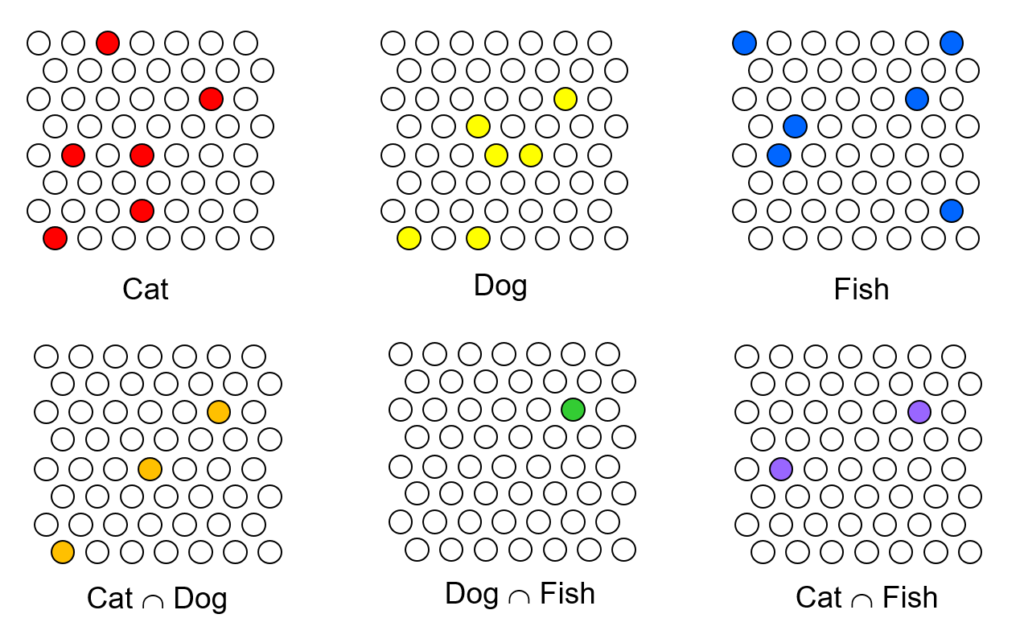This is why I talk about SDC as the coming revolution in computation. Computing the similarities of things is in some sense the essential operation that intelligent computers perform.  Twenty years ago, I demonstrated, in the form of the constructive proof that is my model TEMECOR, now Sparsey®, that choosing an SDC for a new input, which respects the similarity structure of the input space, can be done in fixed time (i.e., the number of steps, thus the compute time and power, remains constant as additional items are added).  In light of the above example, this implies that an SDC system computes an exponential number of similarity relations (of all orders) and reifies them in structure also in fixed-time.

Now, what about the possibility of using localist codes, but not simply placed in an N-space, but stored in a tree structure?  Yes.  This is, I would think, essentially how all modern databases are designed.  The underlying information, the fields of the records, are stored in localist fashion, and some number E of external tree indexes are constructed and point into the records.  Each individual tree index allows finding the best-matching item in the database in log time, but only with respect to the particular query represented by that index.  When a new item is added to the database all E indexes must execute their insertion operations independently.  In the terms used above, each index computes the similarity relations of a new item to ALL N stored items and reifies them using only logN comparisons.  However, the similarities are only those specific to the manifold (subspace) corresponding to index (query).  The total number of similarity relations computed is the sum across the E indexes, as opposed to the product. But it is not this sheer quantitative difference, but rather that having predefined indexes precludes reification of almost all of the similarity relations that in fact may exist and be relevant in the input space.

Thus I claim that SDC admits computing similarity relations exponentially more efficiently than localist coding, even localist codes augmented by external tree indexes.  And, that’s at the heart of why in the future, all intelligent computation will be physically realized via SDC….and why that computation will be able to be done as quickly and power-efficiently as in the brain.